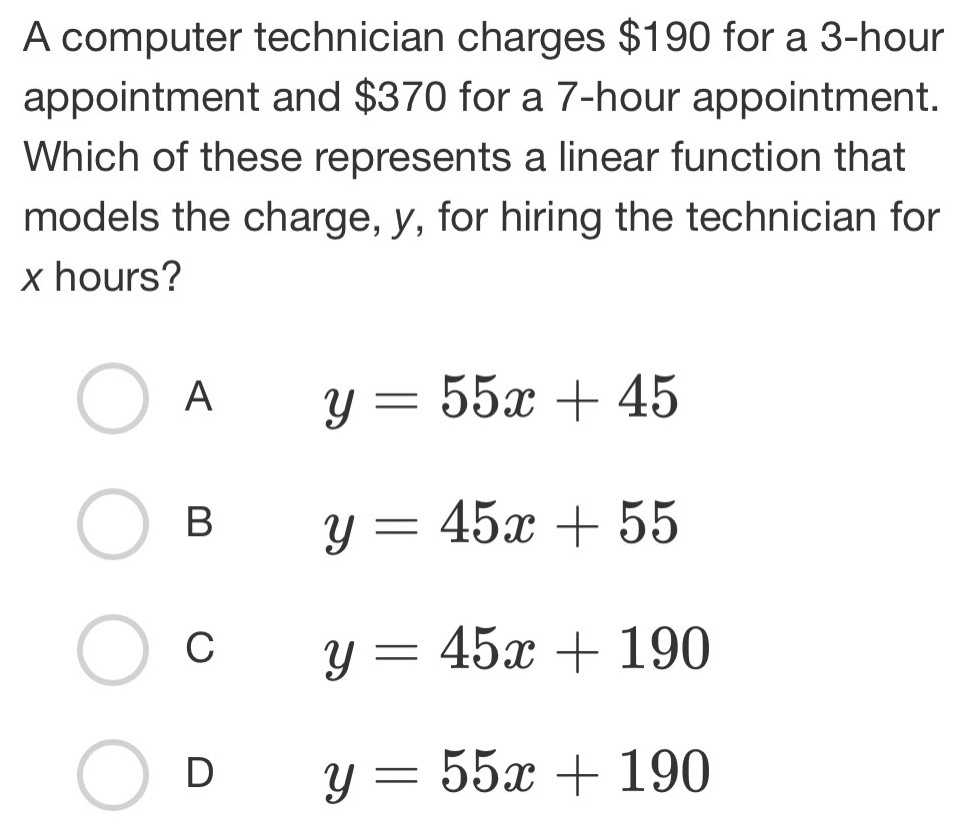### ¿Todavía tienes preguntas de matemáticas?

Pregunte a nuestros tutores expertos
Algebra
PreguntaA computer technician charges $$\ 190$$ for a $$3$$ -hour appointment and $$\ 370$$ for a $$7$$ -hour appointment. Which of these represents a linear function that models the charge, $$y$$ , for hiring the technician for $$x$$ hours?

А $$y = 55 x + 45$$

В $$y = 45 x + 55$$

$$y = 45 x + 190$$

$$y = 55 x + 190$$